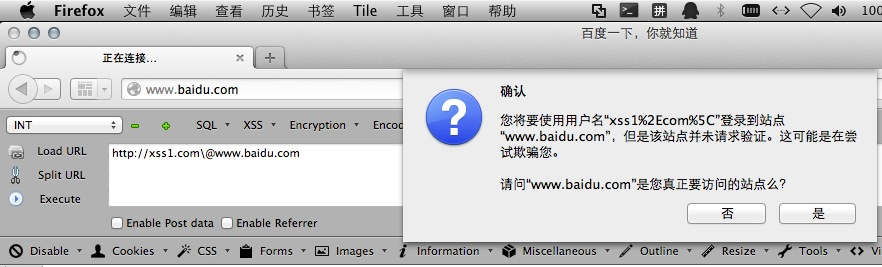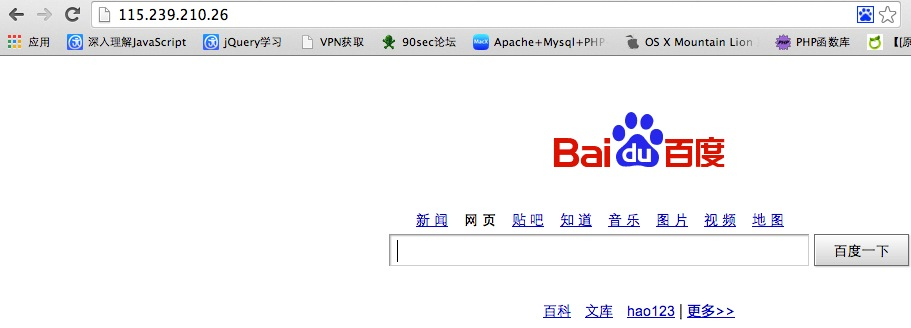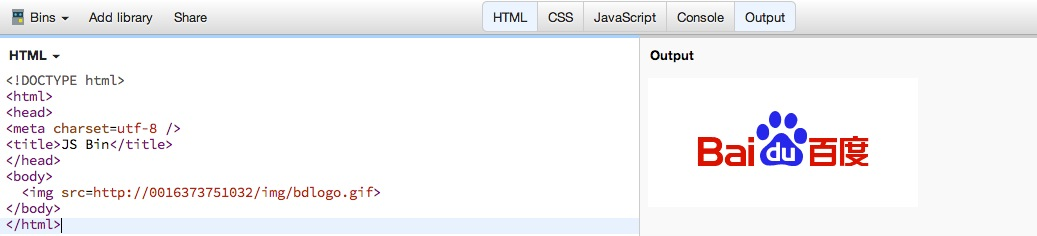## 0x00 目录

``````0x01 链接的构成
0x02 浏览器算如何对url进行解析的
0x03 链接真的只能是这样固定的格式么？
0x04 链接真的是你看到的那样么？
``````

## 0x01 链接的构成

``````第一部分：协议名(以单个冒号结束)

``````

## 0x02 浏览器是如何对url进行解析的

（以下为copy web之困上的内容 他写的比较详细！）

### 3.获取授权信息部分：

``````/(正斜杠)
?(问号)
#(井号)
``````

(1)定位登陆信息，如果有的话：

(2)提取目标地址

``````ftp://admin:[email protected]/* <![CDATA[ */!function(t,e,r,n,c,a,p){try{t=document.currentScript||function(){for(t=document.getElementsByTagName('script'),e=t.length;e--;)if(t[e].getAttribute('data-cfhash'))return t[e]}();if(t&#038;&#038;(c=t.previousSibling)){p=t.parentNode;if(a=c.getAttribute('data-cfemail')){for(e='',r='0x'+a.substr(0,2)|0,n=2;a.length-n;n+=2)e+='%'+('0'+('0x'+a.substr(n,2)^r).toString(16)).slice(-2);p.replaceChild(document.createTextNode(decodeURIComponent(e)),c)}p.removeChild(t)}}catch(u){}}()/* ]]&gt; */:21
``````

ftp协议去登陆主机192.168.1.100，端口号是21端口!

### 例子1：

``````http://xss1.com&[email protected]/* <![CDATA[ */!function(t,e,r,n,c,a,p){try{t=document.currentScript||function(){for(t=document.getElementsByTagName('script'),e=t.length;e--;)if(t[e].getAttribute('data-cfhash'))return t[e]}();if(t&#038;&#038;(c=t.previousSibling)){p=t.parentNode;if(a=c.getAttribute('data-cfemail')){for(e='',r='0x'+a.substr(0,2)|0,n=2;a.length-n;n+=2)e+='%'+('0'+('0x'+a.substr(n,2)^r).toString(16)).slice(-2);p.replaceChild(document.createTextNode(decodeURIComponent(e)),c)}p.removeChild(t)}}catch(u){}}()/* ]]&gt; */
``````

### 例子2：

``````http://xss1.com\@www.baidu.com
``````### 例子3：

``````http://xss1.com;.baidu.com/
``````

## 0x03 链接真的只能是这样固定的格式么？

``````var i='http';
var b='://';
``````

(当前网页的协议是什么 加载这个钩子便用什么协议来加载！ 如在https协议的网页中 这样加载钩子 那么默认就是https去加载钩子了！)``````\\/www.baidu.com
\\@www.baidu.com
\\[email protected]/* <![CDATA[ */!function(t,e,r,n,c,a,p){try{t=document.currentScript||function(){for(t=document.getElementsByTagName('script'),e=t.length;e--;)if(t[e].getAttribute('data-cfhash'))return t[e]}();if(t&#038;&#038;(c=t.previousSibling)){p=t.parentNode;if(a=c.getAttribute('data-cfemail')){for(e='',r='0x'+a.substr(0,2)|0,n=2;a.length-n;n+=2)e+='%'+('0'+('0x'+a.substr(n,2)^r).toString(16)).slice(-2);p.replaceChild(document.createTextNode(decodeURIComponent(e)),c)}p.removeChild(t)}}catch(u){}}()/* ]]&gt; */
\\\\\\\www.baidu.com
///////www.baidu.com
``````www .com .net 什么的特征还在，既然说到猥琐 我们就要更加猥琐！比如下面这样的一串字符串！

``````ⅅʳºℙˢ  -->  drops
ʷººʸⓊⁿ       —>  wooyun
Ⓞʳℊ         —>  org
``````

``````ⅅʳºℙˢ.ʷººʸⓊⁿ.ºʳℊ
``````

http://drops.wooyun.org/papers/146``````DdⅅⅆⅮⅾⒹⓓＤｄ
``````

``````http://ⅅʳºℙˢ.ʷººʸⓊⁿ.ºʳℊ
``````(因为你fuzz的字符多了 会被当成符号 让腾讯认为这不是一个链接！然后就不 能一点就会打开网页了 比如这样。。。)`````` O o º ℴ Ⓞ ⓞ Ｏ ｏ
``````

``````http://laohujijiqiaº8.com
````````````http:[email protected]/* <![CDATA[ */!function(t,e,r,n,c,a,p){try{t=document.currentScript||function(){for(t=document.getElementsByTagName('script'),e=t.length;e--;)if(t[e].getAttribute('data-cfhash'))return t[e]}();if(t&#038;&#038;(c=t.previousSibling)){p=t.parentNode;if(a=c.getAttribute('data-cfemail')){for(e='',r='0x'+a.substr(0,2)|0,n=2;a.length-n;n+=2)e+='%'+('0'+('0x'+a.substr(n,2)^r).toString(16)).slice(-2);p.replaceChild(document.createTextNode(decodeURIComponent(e)),c)}p.removeChild(t)}}catch(u){}}()/* ]]&gt; */#
``````

(ps:以前没加#号时 还是蓝色链接 但是加了#号就显示为腾讯的官网了！)

## 0x04 链接真的是你看到的那样么？

No 任何网站都可以！如下：

``````http:[email protected]/* <![CDATA[ */!function(t,e,r,n,c,a,p){try{t=document.currentScript||function(){for(t=document.getElementsByTagName('script'),e=t.length;e--;)if(t[e].getAttribute('data-cfhash'))return t[e]}();if(t&#038;&#038;(c=t.previousSibling)){p=t.parentNode;if(a=c.getAttribute('data-cfemail')){for(e='',r='0x'+a.substr(0,2)|0,n=2;a.length-n;n+=2)e+='%'+('0'+('0x'+a.substr(n,2)^r).toString(16)).slice(-2);p.replaceChild(document.createTextNode(decodeURIComponent(e)),c)}p.removeChild(t)}}catch(u){}}()/* ]]&gt; */
``````

http:// 后面可以算userinfo 也就算用户信息 账号密码什么的！

[email protected]！ 所以我们这段链接为什么去qq.com 而不是去baidu.com [email protected] 让浏览器认为www.baidu.com 算一段用户信息 而后面的才算主机名 他要去访问的地址！

``````http:[email protected]/* <![CDATA[ */!function(t,e,r,n,c,a,p){try{t=document.currentScript||function(){for(t=document.getElementsByTagName('script'),e=t.length;e--;)if(t[e].getAttribute('data-cfhash'))return t[e]}();if(t&#038;&#038;(c=t.previousSibling)){p=t.parentNode;if(a=c.getAttribute('data-cfemail')){for(e='',r='0x'+a.substr(0,2)|0,n=2;a.length-n;n+=2)e+='%'+('0'+('0x'+a.substr(n,2)^r).toString(16)).slice(-2);p.replaceChild(document.createTextNode(decodeURIComponent(e)),c)}p.removeChild(t)}}catch(u){}}()/* ]]&gt; */
``````

http://0[八进制] 比如 115.239.210.26 首先用.分割数字 115 239 210 26 然后选择10进制转换16进制！

(要用0来表示前缀，可以是一个0也可以是多个0 跟XSS中多加几个0来绕过过滤一样！)

``````首先把这四段数字给 转成 16 进制！结果：73 ef d2 1a  然后把 73efd21a 这十六进制一起转换成8进制！
````````````http://0016373751032
````````````http://xss1.com&[email protected]/* <![CDATA[ */!function(t,e,r,n,c,a,p){try{t=document.currentScript||function(){for(t=document.getElementsByTagName('script'),e=t.length;e--;)if(t[e].getAttribute('data-cfhash'))return t[e]}();if(t&#038;&#038;(c=t.previousSibling)){p=t.parentNode;if(a=c.getAttribute('data-cfemail')){for(e='',r='0x'+a.substr(0,2)|0,n=2;a.length-n;n+=2)e+='%'+('0'+('0x'+a.substr(n,2)^r).toString(16)).slice(-2);p.replaceChild(document.createTextNode(decodeURIComponent(e)),c)}p.removeChild(t)}}catch(u){}}()/* ]]&gt; */
``````

``````http://xss1.com&[email protected]/* <![CDATA[ */!function(t,e,r,n,c,a,p){try{t=document.currentScript||function(){for(t=document.getElementsByTagName('script'),e=t.length;e--;)if(t[e].getAttribute('data-cfhash'))return t[e]}();if(t&#038;&#038;(c=t.previousSibling)){p=t.parentNode;if(a=c.getAttribute('data-cfemail')){for(e='',r='0x'+a.substr(0,2)|0,n=2;a.length-n;n+=2)e+='%'+('0'+('0x'+a.substr(n,2)^r).toString(16)).slice(-2);p.replaceChild(document.createTextNode(decodeURIComponent(e)),c)}p.removeChild(t)}}catch(u){}}()/* ]]&gt; */
``````